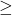## PLS HELP ASAP! Which of the following sets shows all the numbers from the set {0, 0.5, 1, 2.5, 3} that make the inequality 2a + 3 ≥ 8 true?<

Question

PLS HELP ASAP! Which of the following sets shows all the numbers from the set {0, 0.5, 1, 2.5, 3} that make the inequality 2a + 3 ≥ 8 true?

{2.5, 3}
{1, 2.5, 3}
{0, 0.5, 1}
{0.5, 1}

in progress 0
3 months 2021-07-19T22:14:12+00:00 2 Answers 4 views 0

1. The first one: {2.5,3}

first one, [2.5, 3]

Step-by-step explanation:

2a + 38

2a8 – 3

a5/2

a2.5

if we put a =2 .5 in the equation

2a +38

2(2.5) + 38

5+38

88

which is true.

if we put a = 3 in the equation

2a + 38

2(3) +38

6+38

98

which is also true.